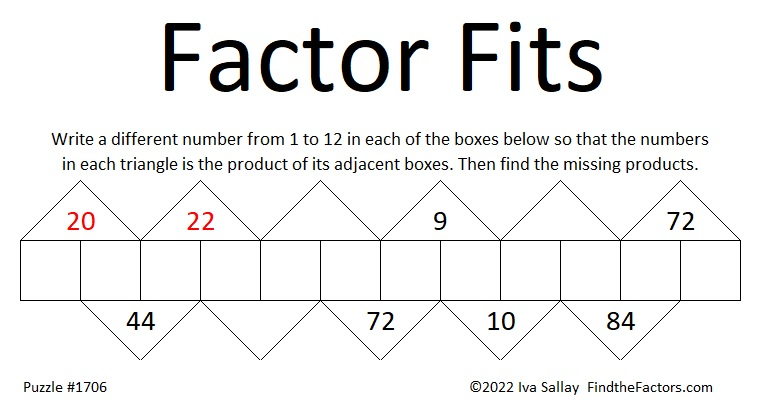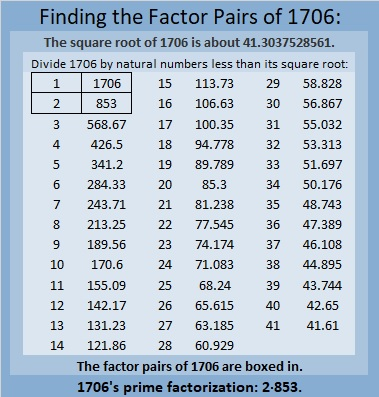# 1706 Can You Make the Factors Fit?

### Today’s Puzzle:

It’s 2022. Happy New Year! I wanted to make a puzzle that has 20 and 22 as clues and thought about what I could do.

I continue to be inspired by an old addition puzzle Sarah Carter @mathequalslove shared on Twitter:

I decided to tweak that puzzle into a multiplication puzzle. I ran into a problem, however. Having products in every triangle made the puzzle way too easy. How do I fix that? I removed some of the product clues. Can you use logic and factoring to know where each factor from 1 to 12 belongs? Can you determine the missing products? I hope you have lots of fun finding the puzzle’s only solution! And I hope you make the factors fit instead of having a fit trying!Here’s something I haven’t told you before: I made lots of multiplication-table puzzles years before I started blogging. I wanted to give the puzzles a good name. At first, I called them “Turn the Tables on Multiplication” or “Turn the Tables” for short. I thought that title was clever but a little bit unwieldy. For a short time, I called the puzzles “Factor Fits.” It was a play on words because all the factors fit, but they might give you fits as you try to find them. I finally settled on “Find the Factors.” That title doubled as instructions for the puzzles. I still liked the name “Factor Fits,” and this puzzle lets me give new life to that name.

### Factors of 1706:

• 1706 is a composite number.
• Prime factorization: 1706 = 2 × 853.
• 1706 has no exponents greater than 1 in its prime factorization, so √1706 cannot be simplified.
• The exponents in the prime factorization are 1 and 1. Adding one to each exponent and multiplying we get (1 + 1)(1 + 1) = 2 × 2 = 4. Therefore 1706 has exactly 4 factors.
• The factors of 1706 are outlined with their factor pair partners in the graphic below.### More About the Number 1706:

1706 is the sum of two squares:
41² + 5² = 1706.

1706 is the hypotenuse of a Pythagorean triple:
410-1656-1706, calculated from 2(41)(5), 41² – 5², 41² + 5².
It is also 2 times (205-828-853).